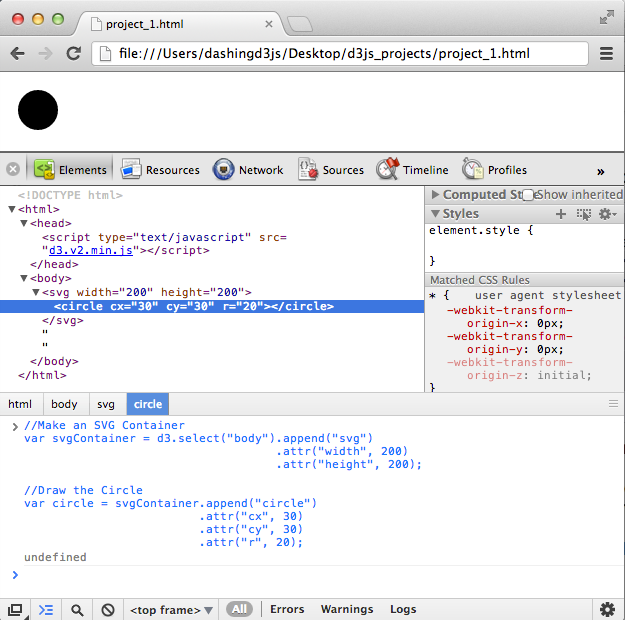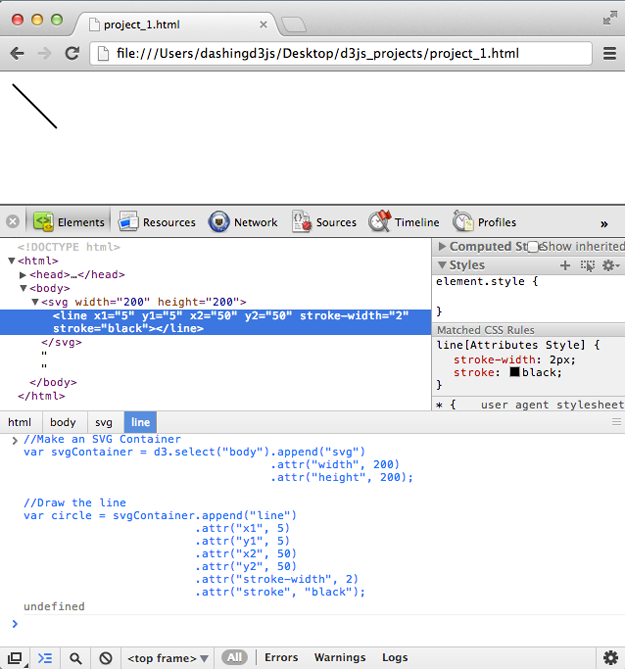# SVG Basic Shapes and D3.js

### The Goal

In this section, we will go over the basic SVG shapes again and how to create them using D3.js.

We will cover previous SVG basic shape examples and how set their attributes using D3.js.

### Drawing an SVG Circle using D3.js

We previously saw how to construct an SVG Circle in the Basic Building Blocks section:

Circle Example & Code:

```1<svg width="50" height="50">
2  <circle cx="25" cy="25" r="25" fill="purple" />
3</svg>```

We have also constructed SVG Circles using D3.js in the previous section (Using JSON to Simplify Code).

``` 1var jsonCircles = [
2  { "x_axis": 30, "y_axis": 30, "radius": 20, "color" : "green" },
3  { "x_axis": 70, "y_axis": 70, "radius": 20, "color" : "purple"},
4  { "x_axis": 110, "y_axis": 100, "radius": 20, "color" : "red"}];
5
6var svgContainer = d3.select("body").append("svg")
7                                    .attr("width", 200)
8                                    .attr("height", 200);
9
10var circles = svgContainer.selectAll("circle")
11                          .data(jsonCircles)
12                          .enter()
13                          .append("circle");
14
15var circleAttributes = circles
16                       .attr("cx", function (d) { return d.x_axis; })
17                       .attr("cy", function (d) { return d.y_axis; })
18                       .attr("r", function (d) { return d.radius; })
19                       .style("fill", function(d) { return d.color; });```

Simplifying the example above, we can then make the process of drawing a circle as two step process:

1) Make an SVG container (the SVG Coordinate Space)

2) Draw the circle

So in the simplest case, our JavaScript code would look like:

``` 1//Make an SVG Container
2var svgContainer = d3.select("body").append("svg")
3                                    .attr("width", 200)
4                                    .attr("height", 200);
5
6//Draw the Circle
7var circle = svgContainer.append("circle")
8                         .attr("cx", 30)
9                         .attr("cy", 30)
10                         .attr("r", 20);```

Which gives us:The necessary SVG attributes for drawing a circle are the "cx", "cy" and "r".

Note - If we leave off the style method, then we get a black circle. Which is fine, we care about making a circle first and foremost, then after that we can style it.

Notice that the important attributes we need to draw an SVG Circle in D3.js are - cx, cy and r.

### Drawing an SVG Rectangle using D3.js

We previously saw how to construct an SVG Rectangle in the Basic Building Blocks section:

Rectangle Example & Code:

```1<svg width="50" height="50">
2  <rect x="0" y="0" width="50" height="50" fill="green" />
3</svg>```

Using the Circle example, you can probably guess that to build a rectangle, you will need an "x", "y", "width" and "height".

You can then make the process of drawing a rectangle as two step process:

1) Make an SVG container (the SVG Coordinate Space)

2) Draw the rectangle

So in the simplest case, our JavaScript code would look like:

``` 1//Make an SVG Container
2var svgContainer = d3.select("body").append("svg")
3                                    .attr("width", 200)
4                                    .attr("height", 200);
5
6//Draw the Rectangle
7var rectangle = svgContainer.append("rect")
8                            .attr("x", 10)
9                            .attr("y", 10)
10                            .attr("width", 50)
11                            .attr("height", 100);```

Which gives us:The necessary SVG attributes for drawing a rectangle are the "x", "y", "width" and "height".

Note - If we leave off the style method, then we get a black rectangle. Which is fine, we care about making a rectangle first and foremost, then after that we can style it.

Two important things to pay attention to

• The SVG word for rectangle is rect. Which makes the append operator .append("rect") not .append("rectangle")!
• The SVG Coordinate system is in place so the height starts from the TOP and moves down

Notice that the important attributes we need to draw an SVG Rectangle in D3.js are - x, y, width and height.

### Drawing an SVG Ellipse using D3.js

We previously saw how to construct an SVG Ellipse in the Basic Building Blocks section:

Ellipse Example & Code:

```1<svg width="50" height="50">
2  <ellipse cx="25" cy="25" rx="15" ry="10" fill="red" />
3</svg>```

Using the Circle and Rectangle examples, you can probably guess that to build an ellipse, you will need a "cx", "cy", "rx" and "ry".

You can then make the process of drawing a ellipse as two step process:

1) Make an SVG container (the SVG Coordinate Space)

2) Draw the ellipse

So in the simplest case, our JavaScript code would look like:

``` 1//Make an SVG Container
2var svgContainer = d3.select("body").append("svg")
3                                    .attr("width", 200)
4                                    .attr("height", 200);
5
6//Draw the Ellipse
7var circle = svgContainer.append("ellipse")
8                         .attr("cx", 50)
9                         .attr("cy", 50)
10                         .attr("rx", 25)
11                         .attr("ry", 10);```

Which gives us:The necessary SVG attributes for drawing an ellipse are the "cx", "cy", "rx" and "ry".

Note - If we leave off the style method, then we get a black ellipse. Which is fine, we care about making a ellipse first and foremost, then after that we can style it.

Notice that the important attributes we need to draw an SVG Ellipse in D3.js are - cx, cy, rx and ry.

### Drawing an SVG Straight Line using D3.js

We previously saw how to construct an SVG Straight Line in the Basic Building Blocks section:

Straight Line Example & Code:

```1<svg width="50" height="50">
2  <line x1="5" y1="5" x2="40" y2="40" stroke="gray" stroke-width="5"  />
3</svg>```

Using the Circle, Rectangle, and Ellipse examples, you can probably guess that to build a straight line, you will need a "x1", "y1", "x2" and "y2".

You can then make the process of drawing a straight line as two step process:

1) Make an SVG container (the SVG Coordinate Space)

2) Draw the straight line

So in the simplest case, our JavaScript code would look like:

``` 1//Make an SVG Container
2var svgContainer = d3.select("body").append("svg")
3                                    .attr("width", 200)
4                                    .attr("height", 200);
5
6//Draw the line
7var circle = svgContainer.append("line")
8                         .attr("x1", 5)
9                         .attr("y1", 5)
10                         .attr("x2", 50)
11                         .attr("y2", 50);```

Which gives us:Wait a second?! Where is the line????? The SVG element is there, however we can't see it in our browser.

It turns out because the SVG ‘line’ elements are single lines and thus are geometrically one-dimensional, that is - they have no interior.

Which means that ‘line’ elements are never filled (see the ‘fill’ property).

Which means that our line does not take up space - so we don't actually see anything.

To fix this, make sure to give the line:

• .attribute("stroke-width", NUMBER), where NUMBER is how wide the line is in units
• .attribute("stroke", "COLOR"), where COLOR is a color to used to color the line

So fixing our simplest case, our JavaScript code would look like:

``` 1        //Make an SVG Container
2var svgContainer = d3.select("body").append("svg")
3                                    .attr("width", 200)
4                                    .attr("height", 200);
5
6//Draw the line
7var circle = svgContainer.append("line")
8                         .attr("x1", 5)
9                         .attr("y1", 5)
10                         .attr("x2", 50)
11                         .attr("y2", 50)
12                         .attr("stroke-width", 2)
13                         .attr("stroke", "black");```

Which gives us:Fantastic - now the line is visible!

The necessary SVG attributes for drawing a straight line are the "x1", "y1", "x2", "y2", "stroke" and "stroke-width".

Note - We don't use a style method with the line. Because ‘line’ elements are single lines and thus are geometrically one-dimensional, they have no interior. Which is why to style them we need to deal with the "stroke" color and "stroke-width".

Notice that the important attributes we need to draw an SVG Straight Line in D3.js are - x1, y1, x2, y2, stroke-width and stroke.

### Drawing Polyline & Polygon SVG Basic Shapes using D3.js

The basic shapes we still have to cover are "Polyline" and "Polygon".

We previously saw how to construct an SVG Polyline and Polygon in the Basic Building Blocks section:

Polyline Example & Code:

``` 1<svg width="50" height="50">
2  <polyline fill="none" stroke="blue" stroke-width="2"
3    points="05,30
4            15,30
5            15,20
6            25,20
7            25,10
8            35,10" />
9</svg>```

Polygon Example & Code:

```1<svg width="50" height="50">
2  <polygon fill="yellow" stroke="blue" stroke-width="2"
3    points="05,30
4            15,10
5            25,30" />
6</svg>```

Using the Circle, Rectangle, Ellipse, and Straight Line examples, you can probably guess that to build a Polyline and Polygon, you will need a "stroke", "stroke-width" and "points". And "fill" for the Polygon.

However, as you can see from the examples above - the points attribute contains a list of points where the x and y are separated by comas and different x and y coordinates are separated by spaces.

Building this out easily in D3.js is not pretty. Because D3.js loves data visualization and pretty things, the D3.js convention is to use the D3.svg.line() generator for polylines and polygons.

To construct the Polyline and Polygon SVG Basic Shapes using D3.js, we will have to learn about SVG Paths.

If you found this D3 Tutorial helpful, you'll enjoy these FREE videos: